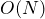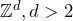# Alexandra Quitmann, Lorenzo Taggi: Macroscopic loops in the Spin O(N) and related models

Abstract: We consider a general system of interacting random loops which includes several models of interest, such as the spinmodel, the double dimer model, random lattice permutations, and is related to the loopmodel and to the interacting Bose gas in discrete space. We consider the system in, and prove the occurrence of macroscopic loops whose length is proportional to the volume of the system as the inverse temperature is large enough. More precisely, we consider arbitrary large boxes and prove that, given any two vertices whose distance is proportional to the diameter of the box, the probability of observing a loop visiting both is uniformly positive.

______________________

Based on

https://arxiv.org/abs/2112.10055

## Video